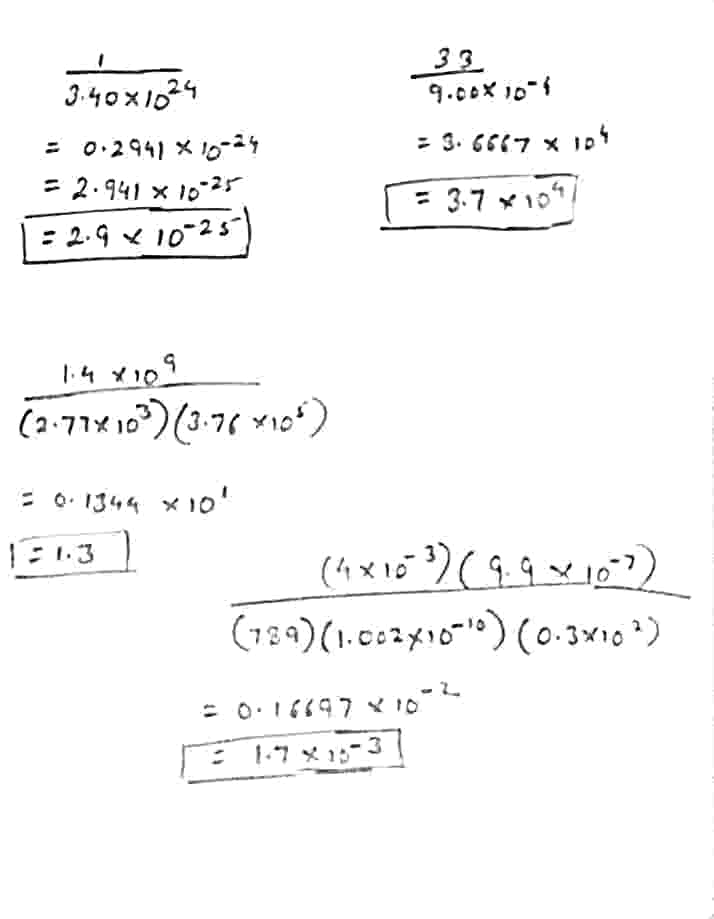# P. Perform each of the following calculations. Round off your answers to two digits

more_vert

4) solve the following problems

P. Perform each of the following calculations. Round off your answers to two digits

a)$$1 \over 3.40 × 10^{24 }$$ ;

b)$$33\over 9.00 × 10^{-4}$$ ;

c) $$1.4 × 10^9\over (2.77 × 10^3 ) (3.76 × 10^5 )$$;

d)$$(4 × 10^{-3}) (9.9 × 10^{-7})\over (789) (1.002 × 10^{-10}\space) (0.3 × 10^2 )$$

more_vert

verified